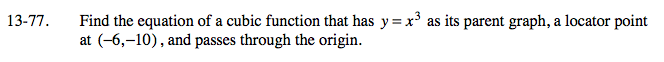### Home > A2C > Chapter 13 > Lesson 13.1.4 > Problem13-77

13-77.Write a general equation based on the locator point:

y = a(x + 6)3 − 10

Substitute (0, 0) for x and y and solve for a.

(0) = a((0) + 6)3 − 10

$y=\frac{10}{216}(x+6)^3-10$